Courses

# RS Aggarwal Test: Pair of Linear Equations in Two Variables

## 10 Questions MCQ Test Mathematics (Maths) Class 10 | RS Aggarwal Test: Pair of Linear Equations in Two Variables

Description
This mock test of RS Aggarwal Test: Pair of Linear Equations in Two Variables for Class 10 helps you for every Class 10 entrance exam. This contains 10 Multiple Choice Questions for Class 10 RS Aggarwal Test: Pair of Linear Equations in Two Variables (mcq) to study with solutions a complete question bank. The solved questions answers in this RS Aggarwal Test: Pair of Linear Equations in Two Variables quiz give you a good mix of easy questions and tough questions. Class 10 students definitely take this RS Aggarwal Test: Pair of Linear Equations in Two Variables exercise for a better result in the exam. You can find other RS Aggarwal Test: Pair of Linear Equations in Two Variables extra questions, long questions & short questions for Class 10 on EduRev as well by searching above.
QUESTION: 1

### If the lines given by 3 x + 2 ky = 2 and 2x + 5y + 1 = 0 are parallel, then value of k is

Solution: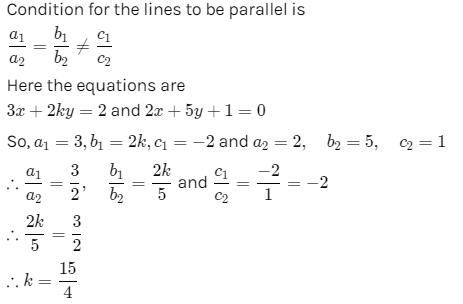QUESTION: 2

### The pair of equations ax + 2y = 7 and 3x + by = 16 represent parallel lines if

Solution: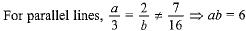QUESTION: 3

### If x = a, y = b is the solution of the equations x - y = 2 and x + y = 4, then the values of a and b are, respectively

Solution:
QUESTION: 4

The equations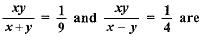equivalent to the equations:

Solution: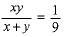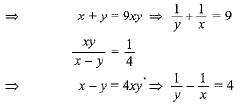QUESTION: 5

The pair of equations 5x - 15y = 8 and 3x - 9y = 24/5 has

Solution:
QUESTION: 6

The value of k for which the system of equations 2x + ky = 12, x + 3y - 4 = 0 are inconsistent is

Solution:
QUESTION: 7

The pair of equations y = 0 and y = -7 has

Solution:
QUESTION: 8

Graphically, the pair of equations 6x - 3y + 10 = 0; 2x - y + 9 = 0 represents two lines which are

Solution:
QUESTION: 9

Five years ago, A was thrice as old as B and ten years later, A shall be twice as old as B. What is the present age of A?

Solution:
QUESTION: 10

One equation of a pair of dependent linear equations is - 5x +7y = 2. The second equation can be

Solution: